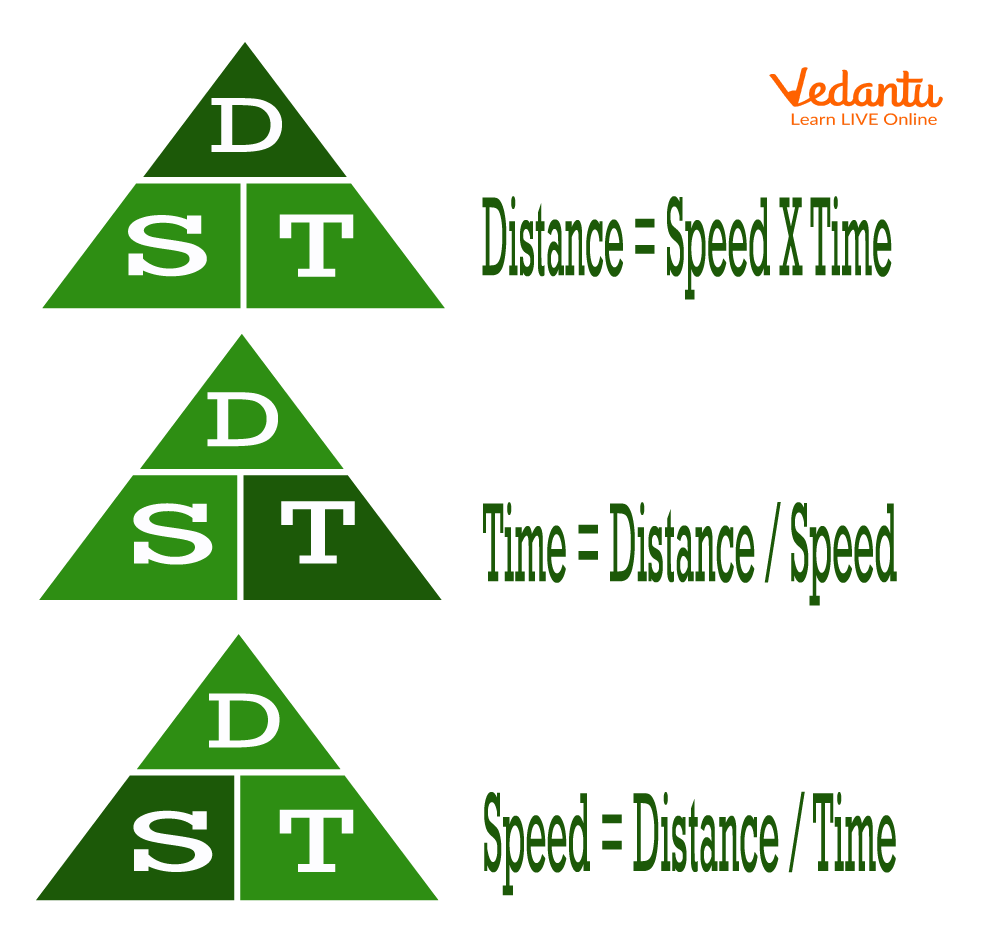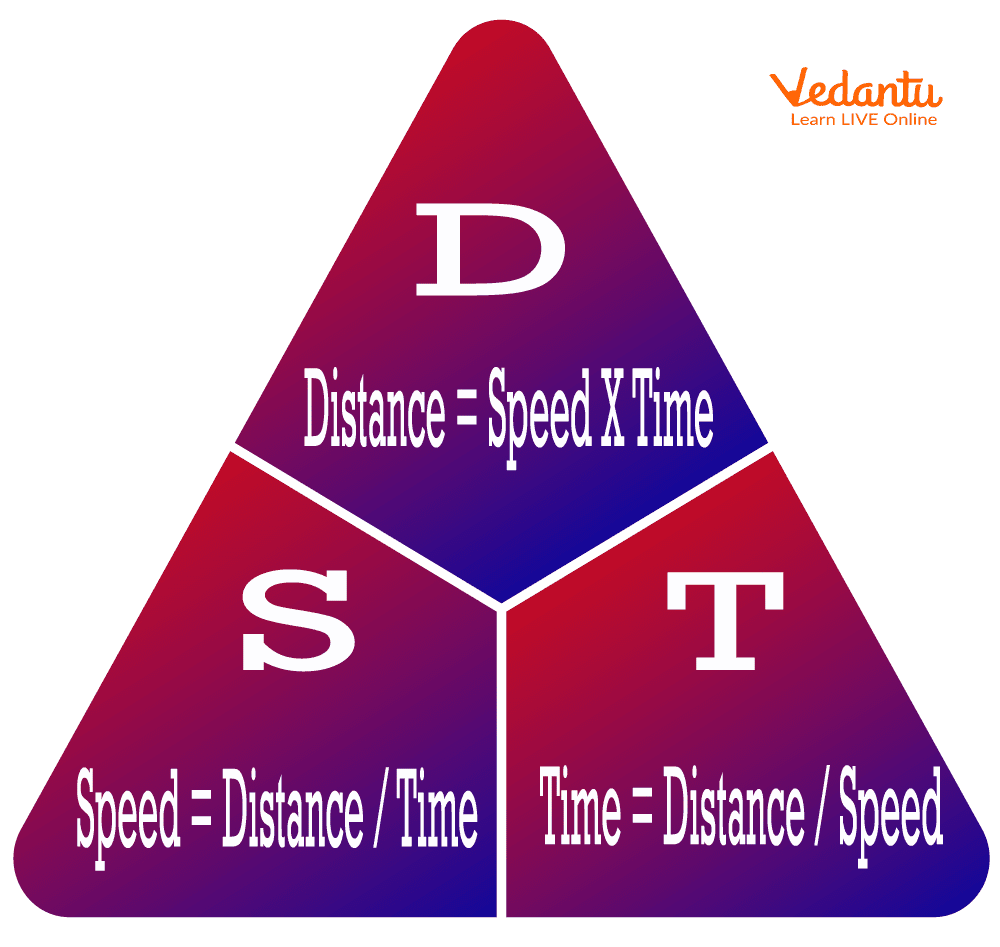Courses
Courses for Kids
Free study material
Free LIVE classes
More

# The Formula of Speed and Its CalculationsLIVE
Join Vedantu’s FREE Mastercalss

## How to Find Speed?

A speed formula represents the distance covered at a specific rate. Distance travelled in a given amount of time is the measure of speed. By knowing the distance travelled by an object and the time it took, you can calculate its average speed. The speed formula and its applications will be discussed in this section.

The formula for speed is distance divided by time. The units of speed are meters per second (m/s) or kilometres per hour (km/hr).

## Deriving the Formula of Speed

Like any other equation, the speed equation = distance /time can be rearranged.

There are three ways to rearrange the formula:

• Distance/time = speed

• Speed* time = distance

• Distance/speed = time

Two variables are needed to compute one (speed, distance, time).Rearrangement of Formula

In the above-given picture, three different triangles are derived from the formula of speed. In the first triangle, the distance (D) is marked as dark green colour, which means that the formula for distance is given. In the second triangle, the time (T) is marked as dark green, which means that the formula for time is given. In the third triangle, the speed (S) is marked as dark green colour, which means the speed calculation formula can be used to find the speed.

## Method for Calculating Average Speed Formula

There is usually a simple formula for calculating the average speed formula.

Speed = $\dfrac{\mathrm{Distance}}{\mathrm{Time}}$

It is possible, however, to use two different speeds for different distances or for different periods of time. It is possible to calculate the average speed using other formulas in these situations. Real-life problems and standardised tests often contain these problems, so learning these formulas and methods is beneficial.

## How Does Speed Affect Time and Distance?

Speed involves both distance and time. "Faster" means either "farther" (greater distance) or "sooner" (less time). Doubling one's speed would mean doubling one's distance travelled in a given amount of time. Doubling one's speed would also mean halving the time required to travel a given distance.Formula in Triangles

## Solved Examples

Example 1: What's your speed if you travel 3600 m in 30 minutes?

Ans: Using the speed calculation formula,

Speed = Distance/ Time

$\dfrac{3600}{30 \times 60}$ = 2m/s = speed

## Method for Measuring Speed

Most Americans measure speed in miles per hour or mph. In most cases, cars are measured by this method. In Physics, meters per second (m/s) is usually used to calculate speed.

### Speed Can be Measured in Two Different Ways:

• Instantaneous Speed - The speed of a particular moment. Depending on how quickly the car is driving at this moment, it might slow down or speed up over the next hour.

• Distance covered by an object over a given time interval is the basis for calculating the average speed. 50 miles travelled in an hour will result in 50 mph. While the car may have been travelling at instantaneous speeds of 40 mph and 60 mph during that period, its average speed was 50 mph.

## Summary

Here we have learnt about the mathematical relation between speed, distance and time. The speed of a moving body is the distance it travels in unit time. A body moving for the same time will travel the same distance.

• Distance = speed x time

• Speed = distance/ time

• To find time, apply the formula for time, t = d/ s, which means time equals distance divided by speed.

• If the distance is in km and time is in hours, then the speed is km/hr.

• If the distance is in m and the time is in seconds, then the speed is m/sec.

Last updated date: 18th Sep 2023
Total views: 109.2k
Views today: 2.09k

## FAQs on The Formula of Speed and Its Calculations

1. How do you use speed in daily life?

When you drive your car, you may do 50 miles per hour, 65 miles per hour, or even 5 miles per hour, depending on traffic. What you see on the speedometer is your instantaneous speed or speed at that instant or particular time. A great example of instantaneous speed is the speed recorded with a radar gun.

2. Why does speed increase distance?

If you keep the speed of an object constant and you increase the weight of that object, it will increase the force of any impact. The faster an object moves, the longer the distance it takes to stop.

3. Cycling 20 kilometres takes 50 minutes. Calculate the cyclist's speed in meters per second using the speed formula.

A cyclist covers 20 meters in a mile by multiplying by 1000

A cyclist takes 50 seconds plus 60 seconds = 3000 seconds

Formulating speed using the formula,

$\dfrac{20000}{3000}$ = 6.67meters/second = speed = distance/time

The cyclist is travelling at a speed of 6.67 m/s.